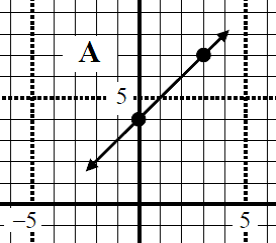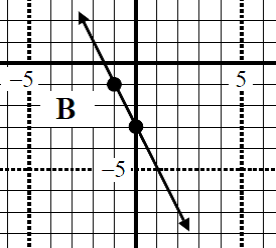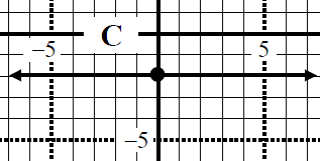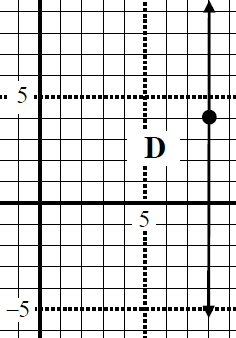# Perpendicular line equation

### Perpendicular line equation

In this lesson, we will look at questions related to perpendicular line equation. We will try to determine perpendicular line equation with different given information such as, graphs, equations of other lines and points.

#### Lessons

• Introduction
a)
How to find the equation of a perpendicular line?

• 1.
Given the graph of linear equation, find the slope of perpendicular line equation.
a)b)c)d)• 2.
The lines 3y + 7x = 3 and cy - 2x - 1 = 0 are perpendicular. Find "c"

• 3.
Determine the equation of a line that is perpendicular to the line 3y + 5x = 8, and passes through the origin. Answer in slope intercept form and general form.